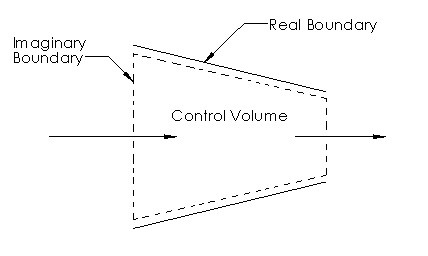# Systems

Systems are defined as a “quantity of matter or a region of space chosen for study.” Anything outside of the system is called the surroundings.  In turn, the surroundings are separated from the system by a boundary.  This boundary can be either real or imaginary.  In addition, the boundary can be fixed or move able.  Also, mathematically the boundary has zero thickness.  This is done to prevent the boundary from containing mass as well as occupying volume.

### Types of Systems

There are two main types of systems; closed or open.

##### Closed System

A close system has a fixed amount of control mass that cannot cross the boundary.  A a result, mass cannot enter or leave the system.  However, energy can cross the boundary as a form of heat or work.  In addition, the volume of a closed system does not need to be fixed.  Instead as energy enters or leaves the system the control volume can expand or contract.  Finally, for certain special cases even energy cannot cross the boundary.  These are known as isolated systems.

The image below shows an example of a closed system.  In this case what is being shown is a piston cylinder device.  Within this device $1~kg$ of air is trapped inside.  As energy is crosses the boundary layer the gas will either expand or contract.  This in turn, will cause a pressure difference that will move the piston either up or down.##### Open System

An open system, also called a control volume, is region in space that has been selected to study.  This type of system can have both real and imaginary surfaces that make up the boundary layer.   Control volumes are used to help define the mass flow through compressors, turbines and nozzles.   Finally, since the system is open, both mass and energy can cross the boundary. This makes it different from a closed system.

When defining a control volume there is no concrete rules on how to define it.  However, depending how the control volume is defined can make a problem easier or harder to solve.  For example, let’s take a look at the image below.  This image represents a nozzle.  When defining the control volume for a nozzle it makes sense to use the inner surfaces of the nozzle as part of the control volume.  The reason why is because we know that the fluid cannot pass through these surfaces.  As a result, now we only have to determine what the mass flow into and out of the nozzle is.Finally, control volumes can be any size or shape.  They can also be fixed or they can have moving boundaries.  However, for simplicity  most control volumes will have fixed boundaries.   Finally, just like with a closed system, heat and work can also interact with a control volume.

##### Properties of a System

A property is used to characterize a certain aspect of the system.  Some common properties are pressure $P$, temperature $T$, volume $V$, and mass $m$.

In addition to being able to define a property you will need to determine if it is intensive or extensive.  For a property to be intensive it must be independent of the mass of system.  Some examples are pressure, density, and temperature.  On the other hand, extensive properties are dependent on the size of the system.  Some of these properties would be total mass, total volume, or total momentum.   To determine if a property is intensive or extensive you can divide the system into two equal parts.  Properties that are intensive will remain the same for both parts.  Properties that are extensive will become half of what they originally were.

Finally, extensive properties per unit mass are called specific properties.  Two examples of specific properties are specific volume $ν$ and specific total energy $e$.  Refer the equations below.

(Eq 1) $e=\frac{E}{m}$

(Eq 2) $ν = \frac{V}{m}$